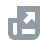Pay with
Markets
NFT
New
English
USDAccount FunctionsTutorialBinance Fan TokenBinance EarnCrypto Deposit/WithdrawalCrypto DerivativesFinanceAPISecurityNFTVIP

2020-07-02 09:52

# .css-1vaed0j{box-sizing:border-box;margin:0;min-width:0;color:#1e2026;}.css-1lohbqv{box-sizing:border-box;margin:0;min-width:0;font-weight:600;}1. Introduction

With Tiered Leverage, the system automatically adjusts the effective leverage based on the amount of user’s borrowed funds, which means that the leverage for an isolated trading pair is dynamic. Thus, the corresponding initial risk ratio and liquidation risk ratio will vary. Herein, there are 10 tiers for 10x isolated margin and 5 tiers for 5x.

# 2. Tiered Leverage Risk Parameters

• 10-level tiered leverage supports up to 10x leverage on Isolated Trading pairs:
• 5-level tiered leverage supports up to 5x leverage on Isolated Trading pairs:

# 3. Borrowing Impact

The rules for borrowing on Isolated Tiered Margin are:
• The greater the amount of funds borrowed, the higher the tiered leverage level; the lower the effective multiple level, the higher the initial and liquidation ratios.
• The smaller the amount of funds borrowed, the lower the tiered leverage level; the higher the effective multiple level, and the lower the initial and liquidation ratios.
• If the user simultaneously borrows the base currency and the quote currency, the system will calculate the new levels separately and the tier will be determined based on whichever is higher.
Using BTC/USDT as an example, the Tiered Leverage are shown in the following table:
• Assuming the user borrowed 15 BTC and 250,000 USDT on Isolated Margin:
• The tier is 4: The borrowed BTC tier is 2 and the borrowed USDT tier is 4. The higher tier is used, which would be tier 4;
• The effective multiple is 7.35 and the corresponding liquidation risk ratio is 1.083;
• Borrowing for VIP users and sub-accounts
• For VIP users and sub-accounts, the system will automatically match the corresponding tier leverage level based on the amount borrowed.

# 4. Tiered Liquidation

Tiered liquidation is subjected to tiered leverage in Isolated Margin.Users’ position will be liquidated partially to match the next tiered leverage if it was under liquidation risk.
• If the tier equals to or greater than 2, and the risk ratio reaches liquidation risk ratio, the system will automatically calculate the amount for the tiered liquidation and reduce the position size to the next one or more tier, until the risk ratio meets the required level.
• Tiered liquidation is carried out in stages, whereby the liquidation engine gradually reduces the positions and calculates the debt to be repaid. If at the next tier the risk ratio is still below the liquidation risk level, partial liquidation will continue until the risk ratio meets the required level.
• In extreme cases, all positions will be liquidated.
Example: Suppose a user borrows 250,000 USDT in the BTCUSDT Isolated Margin account. The effective leverage rate is 7.35, and the corresponding liquidation risk ratio is 1.083.
• Due to market fluctuations, the price of BTC falls, and the user risk ratio is now below 1.083, with the partial liquidation being triggered to repay a debt of 50,000 USDT. The position drops to tier 3, the effective leverage now 8.04, and the corresponding liquidation risk ratio is now at 1.072;
• If the BTC price continues to fall with the risk ratio still below 1.072, the system will continue to perform a gradual liquidation to repay the debt of 80,000 USDT.
By the time the market is stabilized, the user has gone through two rounds of tiered liquidation, and the debt has now become 120,000 USDT. Upon recalculation, the risk ratio is 1.11 (hypothetically) which corresponds to tier 2, with the effective leverage being 8.90, and the liquidation risk ratio being 1.061.

# 5. How to Use Tiered Leverage

• Effective leverage for Isolated Margin can be found here:
• Tier information can be found on the Borrow/Repay pop-up when you borrow
• Isolated margin tiered data can be found here: